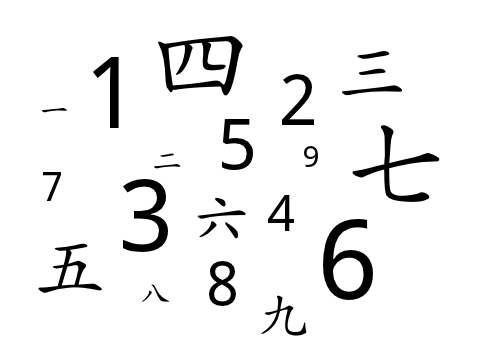Numbers in Chinese
3 years ago
laurasydenham
Save
Edit
Host a game
Live GameLive
Homework
Solo Practice
Practice20 QuestionsShow answers
• Question 1
30 seconds
Q. What is the pinyin for this number 四 ？
sī
sǐ
• Question 2
30 seconds
Q. What is the character for the number 10 ？
• Question 3
30 seconds
Q. What is the English for the number 六？
8
7
6
9
• Question 4
30 seconds
Q. What is the English for the following number 九十八？
98
89
68
88
• Question 5
30 seconds
Q. What is the pinyin for number 5？
wū
wǔ
• Question 6
30 secondsQ.
30
13
10
3
• Question 7
30 seconds
Q. How do you write 88 in characters?
十八八
八十八
八十
八百
• Question 8
30 seconds
Q. What is the pinyin for 七？
chī
qǐ
• Question 9
30 seconds
Q. What is the meaning of this number 五十六？
56
65
5106
57
• Question 10
30 seconds
Q. What is the English for this number 一千？
100
1000
10 000
1 000 000
• Question 11
30 seconds
Q. What is the character for 100
一百
一白
一千
• Question 12
30 seconds
Q. What is the character for 1000
一千
一百
• Question 13
30 seconds
Q. How do you say 四十五 in English?
45
54
4105
• Question 14
30 seconds
Q. What is the English for the following character 六十六?
66
68
6106
6
• Question 15
30 seconds
Q. What is the English for the following character 九十一?
91
92
94
97
• Question 16
30 seconds
Q. What is the character for the number 73?
七十三
六十三
八十三
九十三
• Question 17
30 seconds
Q. What is the number after 六
• Question 18
30 seconds
Q. What is the number before 12?
十一
十二
十三
十四
• Question 19
30 seconds
Q. What is the number before 二十？
十九
十八
二十一
二十三
• Question 20
30 seconds
Q. Which number comes after 九十九？